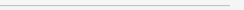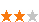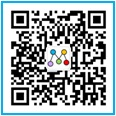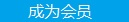您可以捐助，支持我们的公益事业。 1元 10元 50元 认证码：必填求知 文章 文库 Lib 视频 Code iProcess 课程 角色 咨询 工具 火云堂 讲座吧 成长之路要资料订阅捐助
C语言核心之数组和指针详解686 次浏览     评价： 好 中 差

int i=2;

int *p;

p=&i;

 `#include void main() { int a,b,c,*p; a=1; b=3; p=&a; b=*p+1; c=*(p+1); printf("%d %d %d %d /n",a,b,c,*p+3); } `

 `int a={2,4,5,9}; `

 `#include void main() { int a={2,4,5,9}; int *p; p=a; *p=*p++; printf("%d %d %d/n",*p,*p+6,*(p+1)); }`

a[i]表示数组a的第i个元素，如果定义一个指针p，那么语句p=&a;表示可以将指针p指向数组a的第0个元素，也就是说p的值为数组元素a的地址。那么（p+1）引用的是数组元素a的内容，p+i是数组元素a[i]的地址，（p+i）引用的是数组元素a[i]的内容。对数组元素a[i]的引用也可以写成(a+i)。可以得出结论：&a[i]与a+i的含义相同，p[i]与（p+i）也是等价的。

 `int strlen(char *s) { int n; for(n=0;*s!='/0';s++) n++; return n; } `

 `int strlen(char *s) { char *p=s; while(*p!='/0') p++; return p-s; } `

char a[]=”I am a boy”; char *p=”I am a boy”;

a是一个仅仅足以存放初始化字符串以及空字符’/0’的一维数组。数组中的单个字符可以进行修改，但a始终指向同一个存储位置。而p是一个指针，其初值指向一个字符串常量，之后它可以被修改以指向其他地址，但如果试图修改字符串的内容，结果是没有定义的。

 `void strcpy(char *s,char *t) { int i; i=0; while((s[i]=t[i])!='/0') i++; } `

 `void strcpy(char *s,char *t) { while((*s=*t)!='/0'){ s++; t++; } } `

 `void strcpy(char *s,char *t) { while(*s++=*t++) ; } `

 `#include #include void main() { int i; char b[]={"wustrive_2008"}; char *a; *a=b; for(i=0;i

 `#include int strlen(char *s) { char *p=s; while(*p!='/0') p++; return p-s; } void main() { int i; char b[]={"wustrive_2008"}; char *a; *a=b; for(i=0;i

 `int (p)(void ,void *) `

int *f（）; //f是一个函数，它返回一个指向int类型的指针

int (*pf)(); //pf是一个指向函数的指针，该函数返回一个int类型的对象。686 次浏览  评价: 好 中 差订阅捐助

 深度解析：清理烂代码 如何编写出拥抱变化的代码 重构-使代码更简洁优美 团队项目开发"编码规范"系列文章
 重构-改善既有代码的设计 软件重构v2 代码整洁之道 高质量编程规范
 基于HTML5客户端、Web端的应用开发 HTML 5+CSS 开发 嵌入式C高质量编程 C++高级编程

 Visual C++编程命名规则 任何时候都适用的20个C++技巧 C语言进阶 串口驱动分析 轻轻松松从C一路走到C++ C++编程思想

 C++并发处理+单元测试 C++程序开发 C++高级编程 C/C++开发 C++设计模式 C/C++单元测试

 北京 嵌入式C高质量编程 中国航空 嵌入式C高质量编程 华为 C++高级编程 北京 C++高级编程 丹佛斯 C++高级编程 北大方正 C语言单元测试 罗克韦尔 C++单元测试每天2个文档/视频 扫描微信二维码订阅
 订阅技术月刊 获得每月300个技术资源